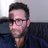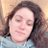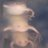## Hole in One: an element reduction approach to modeling bone porosity in finite element analysisRT @Tseng_ZJ: A "Hole in One"! @BeatricheGPS's thesis preprint has been published today in @PeerJPreprints https://t.co/A1jYM1yms4 #Computa…30 days ago
RT @Tseng_ZJ: A "Hole in One"! @BeatricheGPS's thesis preprint has been published today in @PeerJPreprints https://t.co/A1jYM1yms4 #Computa…My preprint has been published today in @PeerJPreprints https://t.co/jaUclgKHdb #ComputationalBiology #EvolutionaryStudies #PaleontologyRT @Tseng_ZJ: A "Hole in One"! @BeatricheGPS's thesis preprint has been published today in @PeerJPreprints https://t.co/A1jYM1yms4 #Computa…A "Hole in One"! @BeatricheGPS's thesis preprint has been published today in @PeerJPreprints https://t.co/A1jYM1yms4 #ComputationalBiology #EvolutionaryStudies #Paleontology31 days ago
Hole in One: an element reduction approach to modeling bone porosity in finite element analysis https://t.co/9HeZzZgEob https://t.co/uchVO0eLVsHole in One: an element reduction approach to modeling bone porosity in finite element analysis https://t.co/MBV9aSGOyh https://t.co/Ie0Eats6Vd
NOT PEER-REVIEWED
"PeerJ Preprints" is a venue for early communication or feedback before peer review. Data may be preliminary.

## Supplemental Information

### Scan numbers and filled volume of the CC

The high-resolution CT-scans were obtained with the GE v|tome|x s scanner at the American Museum of Natural History (AMNH).

### Code performance consistency

1 to 10 points refer to the sampled points. Being 1 at the top area of the cylinder and 10 at the bottom. I to V refer to the five coded modified cylinders. Beneath those values, we present some statistical information (Mean: average; Stdev: standard deviation; Sterr: standard error of the mean; 95% CI: Confident Interval at 95 percent).

### von Mises stress values and statistical analysis

1 to 10 points refers to the sampled points. Being 1 at the top area of the cylinder and 10 at the bottom. T1 to T4 refers to each transect (left column stress in MPa; right column node number). The final four columns present some statistical information (Mean: average; Stdev: standard deviation; Sterr: standard error of the mean; 95% CI: Confident Interval at 95 percent).

### von Mises stress values and statistical analysis

1 to 10 points refers to the sampled points. Being 1 at the top area of the cylinder and 10 at the bottom. T1 to T4 refers to each transect (left column stress in MPa; right column node number). The final four columns present some statistical information (Mean: average; Stdev: standard deviation; Sterr: standard error of the mean; 95% CI: Confident Interval at 95 percent).

### von Mises stress values and statistical analysis

1 to 10 points refers to the sampled points. Being 1 at the top area of the cylinder and 10 at the bottom. T1 to T4 refers to each transect (left column stress in MPa; right column node number). The final four columns present some statistical information (Mean: average; Stdev: standard deviation; Sterr: standard error of the mean; 95% CI: Confident Interval at 95 percent).

### von Mises stress values and statistical analysis

1 to 10 points refers to the sampled points. Being 1 at the top area of the cylinder and 10 at the bottom. T1 to T4 refers to each transect (left column stress in MPa; right column node number). The final four columns present some statistical information (Mean: average; Stdev: standard deviation; Sterr: standard error of the mean; 95% CI: Confident Interval at 95 percent).

### von Mises stress values and statistical analysis

1 to 10 points refers to the sampled points. Being 1 at the top area of the cylinder and 10 at the bottom. T1 to T4 refers to each transect (left column stress in MPa; right column node number). The final four columns present some statistical information (Mean: average; Stdev: standard deviation; Sterr: standard error of the mean; 95% CI: Confident Interval at 95 percent).

### von Mises stress values and statistical analysis

1 to 10 points refers to the sampled points. Being 1 at the top area of the cylinder and 10 at the bottom. T1 to T4 refers to each transect (left column stress in MPa; right column node number). The final four columns present some statistical information (Mean: average; Stdev: standard deviation; Sterr: standard error of the mean; 95% CI: Confident Interval at 95 percent).

### von Mises stress values and statistical analysis

1 to 10 points refers to the sampled points. Being 1 at the top area of the cylinder and 10 at the bottom. T1 to T4 refers to each transect (left column stress in MPa; right column node number). The final four columns present some statistical information (Mean: average; Stdev: standard deviation; Sterr: standard error of the mean; 95% CI: Confident Interval at 95 percent).

### von Mises stress values and statistical analysis

1 to 10 points refers to the sampled points. Being 1 at the top area of the cylinder and 10 at the bottom. T1 to T4 refers to each transect (left column stress in MPa; right column node number). The final four columns present some statistical information (Mean: average; Stdev: standard deviation; Sterr: standard error of the mean; 95% CI: Confident Interval at 95 percent).

### von Mises stress values and statistical analysis

1 to 10 points refers to the sampled points. Being 1 at the top area of the cylinder and 10 at the bottom. T1 to T4 refers to each transect (left column stress in MPa; right column node number). The final four columns present some statistical information (Mean: average; Stdev: standard deviation; Sterr: standard error of the mean; 95% CI: Confident Interval at 95 percent).

### von Mises stress values and statistical analysis

1 to 10 points refers to the sampled points. Being 1 at the top area of the cylinder and 10 at the bottom. T1 to T4 refers to each transect (left column stress in MPa; right column node number). The final four columns present some statistical information (Mean: average; Stdev: standard deviation; Sterr: standard error of the mean; 95% CI: Confident Interval at 95 percent).

### von Mises stress values and statistical analysis

1 to 10 points refers to the sampled points. Being 1 at the top area of the cylinder and 10 at the bottom. T1 to T4 refers to each transect (left column stress in MPa; right column node number). The final four columns present some statistical information (Mean: average; Stdev: standard deviation; Sterr: standard error of the mean; 95% CI: Confident Interval at 95 percent).

### von Mises stress values and statistical analysis

1 to 10 points refers to the sampled points. Being 1 at the top area of the cylinder and 10 at the bottom. T1 to T4 refers to each transect (left column stress in MPa; right column node number). The final four columns present some statistical information (Mean: average; Stdev: standard deviation; Sterr: standard error of the mean; 95% CI: Confident Interval at 95 percent).

### von Mises stress values and statistical analysis

1 to 10 points refers to the sampled points. Being 1 at the top area of the cylinder and 10 at the bottom. T1 to T4 refers to each transect (left column stress in MPa; right column node number). The final four columns present some statistical information (Mean: average; Stdev: standard deviation; Sterr: standard error of the mean; 95% CI: Confident Interval at 95 percent).

### von Mises stress values and statistical analysis

1 to 10 points refers to the sampled points. Being 1 at the top area of the cylinder and 10 at the bottom. T1 to T4 refers to each transect (left column stress in MPa; right column node number). The final four columns present some statistical information (Mean: average; Stdev: standard deviation; Sterr: standard error of the mean; 95% CI: Confident Interval at 95 percent).

### von Mises stress values and statistical analysis

1 to 10 points refers to the sampled points. Being 1 at the top area of the cylinder and 10 at the bottom. T1 to T4 refers to each transect (left column stress in MPa; right column node number). The final four columns present some statistical information (Mean: average; Stdev: standard deviation; Sterr: standard error of the mean; 95% CI: Confident Interval at 95 percent).

### von Mises stress values and statistical analysis

1 to 10 points refers to the sampled points. Being 1 at the top area of the cylinder and 10 at the bottom. T1 to T4 refers to each transect (left column stress in MPa; right column node number). The final four columns present some statistical information (Mean: average; Stdev: standard deviation; Sterr: standard error of the mean; 95% CI: Confident Interval at 95 percent).

### von Mises stress values and statistical analysis

1 to 10 points refers to the sampled points. Being 1 at the top area of the cylinder and 10 at the bottom. T1 to T4 refers to each transect (left column stress in MPa; right column node number). The final four columns present some statistical information (Mean: average; Stdev: standard deviation; Sterr: standard error of the mean; 95% CI: Confident Interval at 95 percent).

### von Mises stress values and statistical analysis

1 to 10 points refers to the sampled points. Being 1 at the top area of the cylinder and 10 at the bottom. T1 to T4 refers to each transect (left column stress in MPa; right column node number). The final four columns present some statistical information (Mean: average; Stdev: standard deviation; Sterr: standard error of the mean; 95% CI: Confident Interval at 95 percent).

### von Mises stress values and statistical analysis

1 to 10 points refers to the sampled points. Being 1 at the top area of the cylinder and 10 at the bottom. T1 to T4 refers to each transect (left column stress in MPa; right column node number). The final four columns present some statistical information (Mean: average; Stdev: standard deviation; Sterr: standard error of the mean; 95% CI: Confident Interval at 95 percent).

### Competing Interests

The authors declare that they have no competing interests.

### Author Contributions

Beatriz L Santaella conceived and designed the experiments, performed the experiments, analyzed the data, prepared figures and/or tables, authored or reviewed drafts of the paper, approved the final draft.

Z. Jack Tseng conceived and designed the experiments, analyzed the data, contributed reagents/materials/analysis tools, authored or reviewed drafts of the paper, approved the final draft.

### Data Deposition

The following information was supplied regarding data availability:

Data is available at GITHUB, as ~BeaSantaella/Hole-in-One https://github.com/BeaSantaella/Hole-in-One

Data is available at Zendodo, as ~Hole in One https://doi.org/10.5281/zenodo.3344501

### Funding

B. Santaella was funded by a research scholarship from the Functional Anatomy and Vertebrate Evolution Laboratory. The funders had no role in study design, data collection and analysis, decision to publish, or preparation of the manuscript.

Some Markdown syntax is allowed: _italic_ **bold** ^superscript^ ~subscript~ %%blockquote%% [link text](link URL)